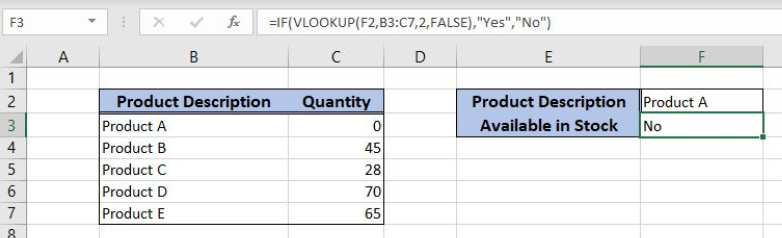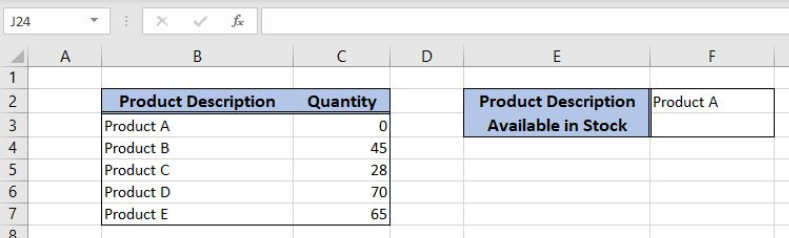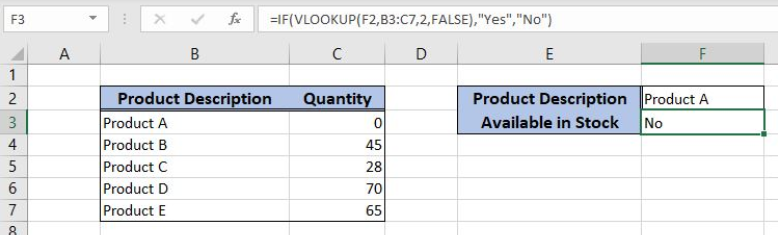Get instant live expert help with Excel or Google Sheets“My Excelchat expert helped me in less than 20 minutes, saving me what would have been 5 hours of work!”

#### Post your problem and you’ll get expert help in seconds.

Your message must be at least 40 characters
Our professional experts are available now. Your privacy is guaranteed.

# Here is How You Can Combine an IF Statement and a Lookup Function in Excel

Excel allows a user to combine the IF statement and the VLOOKUP functions. This step by step tutorial will assist all levels of Excel users to learn how to combine an IF statement and a lookup function in Excel.Figure 1. The result of the formula

## Syntax of the VLOOKUP formula

The generic formula for the VLOOKUP function is:

`=VLOOKUP(lookup_value, table_array, col_index_num, range_lookup)`

The parameters of the VLOOKUP function are:

• lookup_value – a value that we want to find in a table_array
• table_array – a range in which we want to lookup
• col_index_num – a column number in table_array from which we would like to get a value
• range_lookup – default value is FALSE. This means that we want to find an exact match for a lookup value.

## Syntax of the IF Formula

The generic formula for the IF function is:

`=IF(logical_test, value_if_true, value_if_false)`

The parameters of the IF function are:

• logical_test – a logical expression that we want to check
• value_if_true – a value which the function returns if a logical_test is TRUE
• value_if_false – a value which the function returns if a logical_test is FALSE.

## Setting up Our Data for the Formula

Let’s look at the structure of the data. In the range B2:C7 we have a table from which we want to pull data. The table has two columns “Product Description” (column B) and “Quantity” (column C). In the cell F2, we have the product which we want to check. In F3, we want to to get “Yes” if the quantity of the product is greater than 0, or “No” if it’s 0.Figure 2. The data structure for the formula

## Check if a Product is Out of the Stock Using the Formula

In this example, we want to check the quantity of “Product A”. If the quantity is 0, we will return “No” in F3 and if it’s greater than 0, we will return “Yes”.

The formula looks like:

`=IF(VLOOKUP(F2, B3:C7, 2, FALSE), "Yes", "No")`

In our example, the lookup_value is the F2 cell (“Product A”). The parameter table_array is B3:C7 because we want to find value from the range B3:B7. Col_index_num has value 2, as we want to pull value from the second column of the range. Finally, range_lookup has value FALSE, because we want to find an exact match of “Product ID” values. The result of the VLOOKUP function is the logical_test of the IF function. The value_if_true is “Yes” and the value_if_false is “No”.

To apply the VLOOKUP function, we need to follow these steps:

• Select cell F3 and click on it
• Insert the formula: `=IF(VLOOKUP(F2, B3:C7, 2, FALSE), "Yes", "No")`
• Press enterFigure 3. Using the formula to check if the product is out of the stock

The quantity for Product A in the cell C3 is 0. Therefore, the VLOOKUP function returns 0. This is considered as false logical condition, so the result in the cell F3 is “No”.

Most of the time, the problem you will need to solve will be more complex than a simple application of a formula or function. If you want to save hours of research and frustration, try our live Excelchat service! Our Excel Experts are available 24/7 to answer any Excel question you may have. We guarantee a connection within 30 seconds and a customized solution within 20 minutes.

Solution examplesI filtered data in column A (it is labeled ID #) of a data set. On my next worksheet, column A is also the ID #, but it is a different data set. I want to filter the ID #'s the same for the two sheets, but how do I do that being that I am working with two different sets of data?
Solved by V. H. in 22 minsCould you help me by explaining the IF function?
Solved by G. L. in 24 minsVLOOKUP help. Trying to input data from database into new table
Solved by S. Q. in 40 minsI'm looking for a formula or format for if there is data in c4 on a sheet... It adds extra information underneath
Solved by X. E. in 20 minshi, my vlookup wont work in some rows but does in others. its temperemental. e.g it will work on rows 10 to 15, then not row 16 and 17, but the will for the rest.
Solved by K. Q. in 21 mins